﻿ 采用相关滤波的水下海参目标跟踪
«上一篇文章快速检索 高级检索

 智能系统学报2019, Vol. 14Issue (3): 525-532  DOI: 10.11992/tis.2017110370

### 引用本文LIU Jiwei, WEI Honglei, PEI Qichao, et al. Underwater sea cucumber target tracking algorithm based on correlation filtering[J]. CAAI Transactions on Intelligent Systems, 2019, 14(3): 525-532. DOI: 10.11992/tis.201711037.### 文章历史

1. 大连工业大学 机械工程与自动化学院，辽宁 大连 116034;
2. 华北理工大学 机械学院，河北 唐山 063210

Underwater sea cucumber target tracking algorithm based on correlation filtering
LIU Jiwei 1, WEI Honglei 1, PEI Qichao 1, XING Liran 2
1. Institute of Mechanical Engineering and Automation, Dalian Polytechnic University, Dalian 116034, China;
2. College of Mechanical Engineering, North China University of Science and Technology, Tangshan 063210, China
Abstract: This study proposes a type of sea cucumber target tracking algorithm based on the kernel correlation filter (KCF) to find a solution for real-time tracking while capturing a sea cucumber using an underwater robot. In the initial frame, the image block that contains the target sea cucumber is divided into nine sub-blocks based on the characteristics of the sea cucumber, including its appearance and the positioning of its two heads by comparing the boundary blocks with the central block. Further, the KCF algorithm is used to track the two heads of the sea cucumber in the subsequent frames, estimate the scale, and calculate the location of the sea cucumber based on the distance variation between the two modules . The experimental results exhibit that the accuracy, running speed, and success rate of the tracking algorithm are higher than those of other experimental algorithms.
Key words: visual tracking    circulant matrices    discrete Fourier transform    kernel methods    ridge regression    correlation filters    capturing sea cucumbers    scale estimation

1 KCF跟踪方法

KCF算法(Kernel correlation filters)通过核化岭回归分析方法解决目标追踪问题。在相关滤波方法的基础上，利用基准样本的循环位移方法构造训练集训练分类器，而且在训练和检测时利用快速傅里叶变换将耗时的矩阵运算转换到频域求解，显著提高了跟踪精度和效率。

1.1 循环矩阵

 ${{X}} = {{C}}({{x}}) = \left[ {\begin{array}{*{20}{c}} {{x_1}}&{{x_2}}&{{x_3}}& \cdots &{{x_n}} \\ {{x_n}}&{{x_1}}&{{x_2}}& \cdots &{{x_{n - 1}}} \\ {{x_{n - 1}}}&{{x_n}}&{{x_1}}& \cdots &{x{}_{n - 2}} \\ \vdots & \vdots & \vdots & & \vdots \\ {{x_2}}&{{x_3}}&{{x_4}}& \cdots &{{x_1}} \end{array}} \right]$ (1)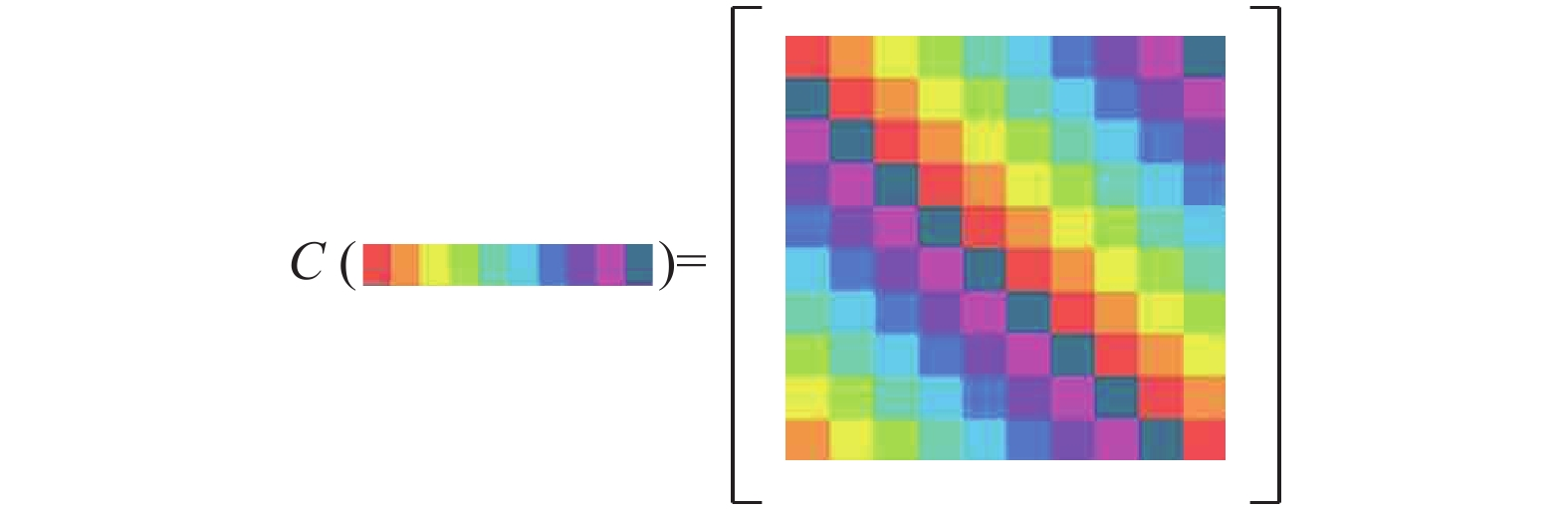Download: 图 1 循环矩阵 Fig. 1 Illustration of a circulant matrix

 ${{C}}({{x}}) = {{{F}}^{\rm{H}}}{\rm{diag}}\left( {\hat {{x}}} \right){{F}}$ (2)

 $\left\{ \begin{array}{l} \hat {{x}} = {{F}}{{x}} \\ {{{F}}^ * } = {{{F}}^{\rm{H}}} = {{{F}}^{ - 1}} \\ {{F}} = {{{F}}^{\rm{T}}} \\ \end{array} \right.$ (3)
1.2 训练样本的建立

 ${y_{ij}} = \exp \,\, \left( - \frac{{{{(i - w)}^2} + {{(j - h)}^2}}}{{2\delta _p^2}}\right)$ (4)Download: 图 2 基础输出样本 Fig. 2 Basic output sample

$(x,y)$ 所有循环移位得到的图像块 $({x_i},{y_i})$ 作为训练样本，如图3所示。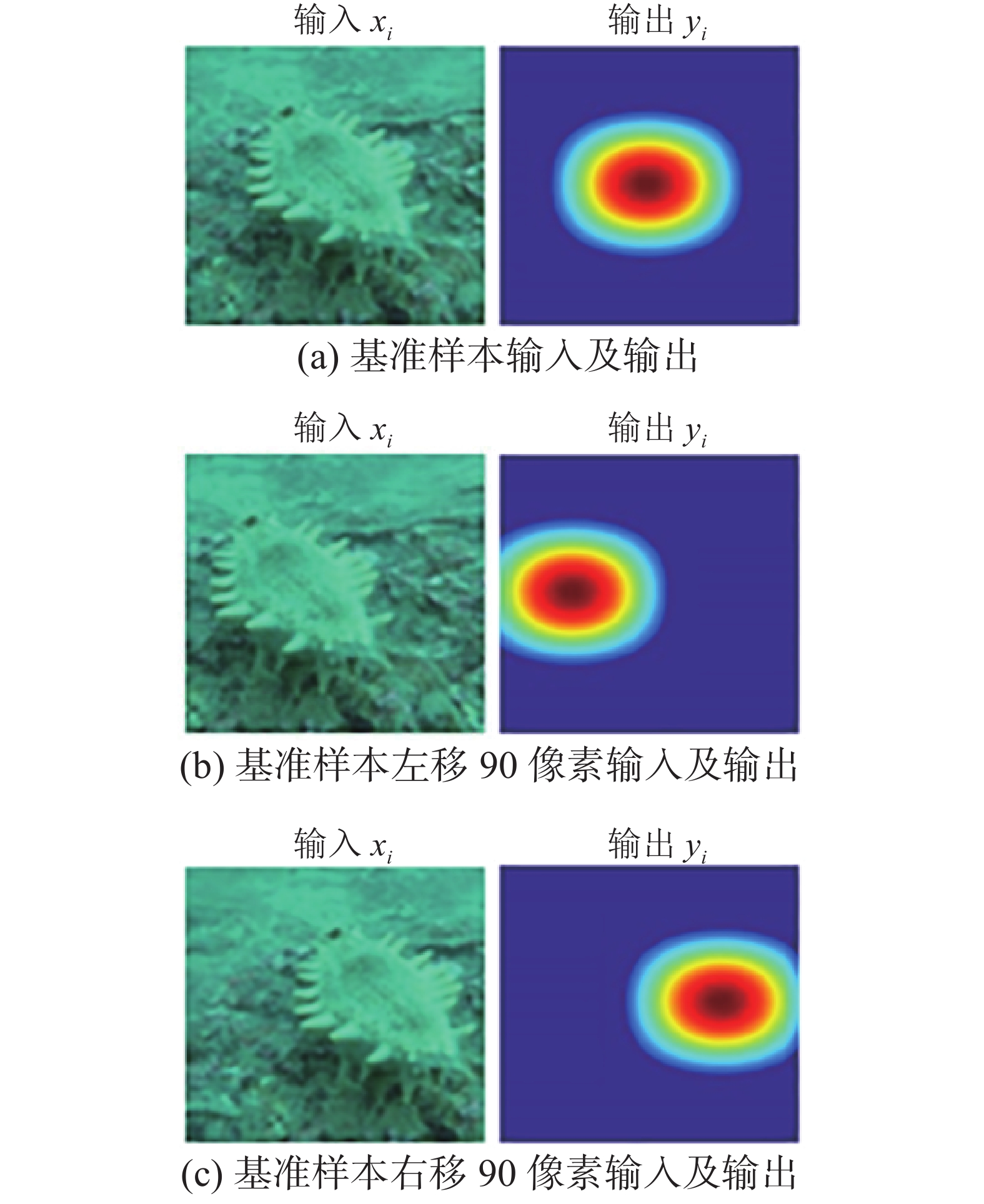Download: 图 3 利用基础样本的循环移位方法示例 Fig. 3 Examples depicting the cyclic shifts of a base sample
1.3 跟踪算法

KCF算法的基本流程分为离线训练和在线检测两步。在训练时，以初始帧中给出的目标图像为输入信息，对应的高斯响应为输出信息，利用离散傅里叶变换转换到频域求解滤波器。在线跟踪检测时，用滤波器对包含目标的图像进行滤波可得到高斯型的响应面。响应面最大的位置就是目标位置。KCF算法的基本流程如图4所示。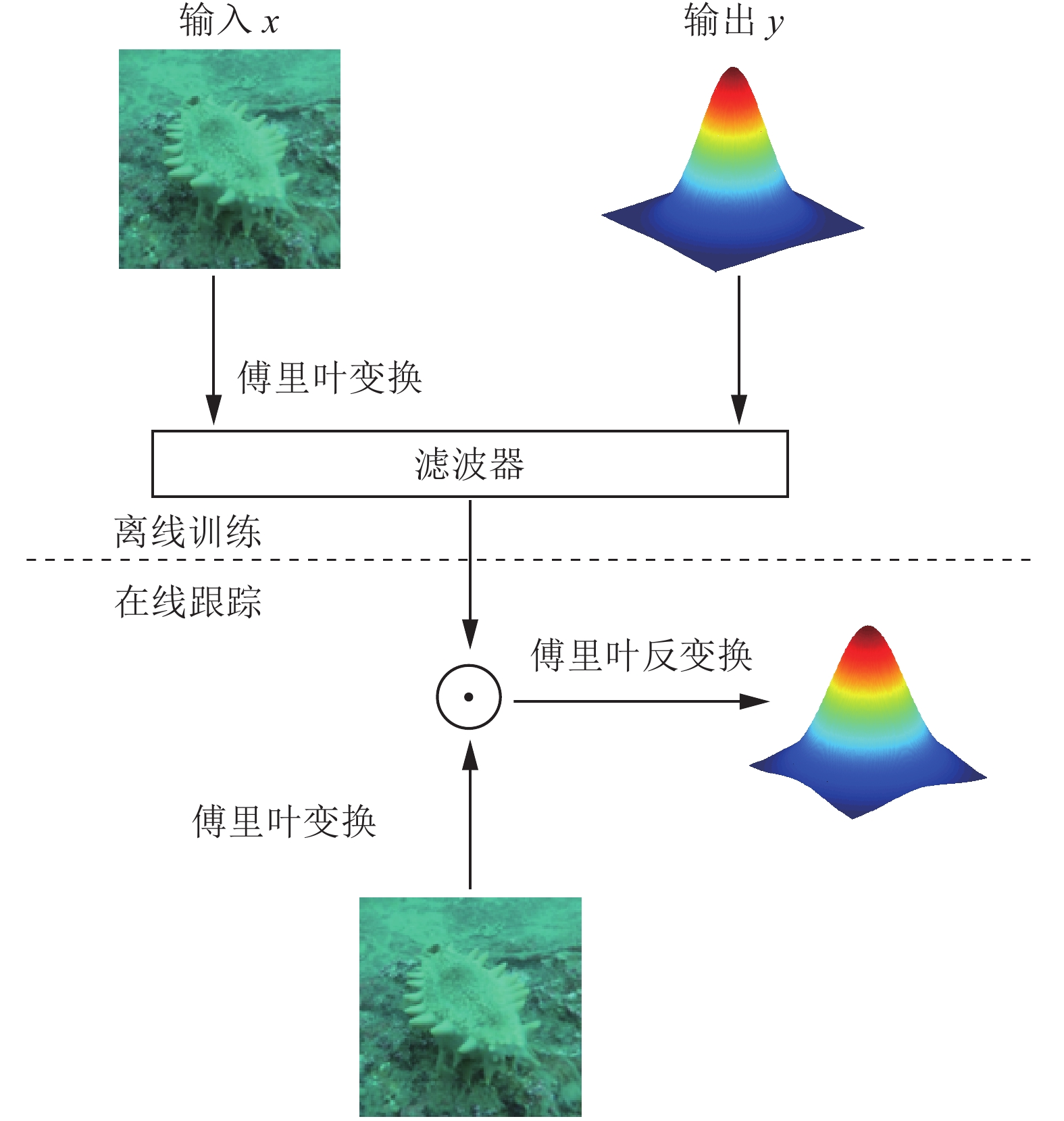Download: 图 4 KCF算法的基本流程 Fig. 4 Schematic representation of the KCF method

 $\mathop {{\rm{min}}}\limits_w {\sum\limits_i {{{(f({x_i}) - {y_i})}^2} + {\textit{λ}} \left\| w \right\|} ^2}$ (5)

 ${{w}} = {{{X}}^{\rm{T}}}{({{X}}{{{X}}^{\rm{T}}} +{\textit{λ}} {{I}})^{ - 1}}{{Y}}$ (6)

 ${{\alpha }} = {({{K}} + {\textit{λ}} {{I}})^{ - 1}}{{Y}}$ (7)

 ${{w}} = {{{X}}^{\rm{T}}}\alpha = \sum\limits_{i = 1}^n {{\alpha _i}{x_i}}$ (8)

 ${\hat \alpha ^ * } = \frac{{\hat {{y}}}}{{\hat \kappa ({{x}},{{x}}) + {\textit{λ}} }}$ (9)

 $\hat \kappa \left( {{{x}},{{x}}'} \right) = \exp \left( { - \frac{1}{{{\sigma ^2}}}{{\left( {\left\| {{x}} \right\|} \right)}^2} + {{\left( {\left\| {{{x}}'} \right\|} \right)}^2} - 2{F^{ - 1}}\left( {\hat {{x}} \odot \hat {{x}}'*} \right)} \right)$ (10)

 \begin{aligned} f({{z}}) = & {\left( {\sum\limits_{i = 1}^n {{{{\alpha}} _i}{{\phi}} ({{{x}}_i})} } \right)^{\rm{T}}}{{\phi}} ({{{{z}}}}) = \sum\limits_{i = 1}^n {{{{\alpha}} _i}{{{\phi}} ^{\rm{T}}}({{{x}}_i})} {{\phi}} ({{{{z}}}}) = \\ & \sum\limits_{i = 1}^n {{{{\alpha}} _i}\kappa ({{{x}}_i},{{{{z}}}})} = {({{C}}(\kappa ({{x}},{{{{z}}}})))^{\rm{T}}}{{\alpha}} \end{aligned} (11)

 $f = {{{F}}^{ - 1}}\left( {\hat \kappa {{({{x}},{{{{z}}}})}^ * } \odot \hat {{\alpha}} } \right)$ (12)

 ${\hat {{\alpha}} _{t + 1}} = \left( {1 - \beta } \right){\hat {{\alpha}} _{t - 1}} + \beta {\hat {{\alpha}} _t}$ (13)

 ${\hat {{x}}_{t + 1}} = \left( {1 - \beta } \right){\hat {{x}}_{t - 1}} + \beta {\hat {{x}}_t}$ (14)

2 改进算法

2.1 海参头部定位

 $({x_A},{x_B}) \in \left\{ {({x_{11}},{x_{33}}),({x_{12}},{x_{32}}),({x_{13}},{x_{31}}),({x_{21}},{x_{23}})} \right\}$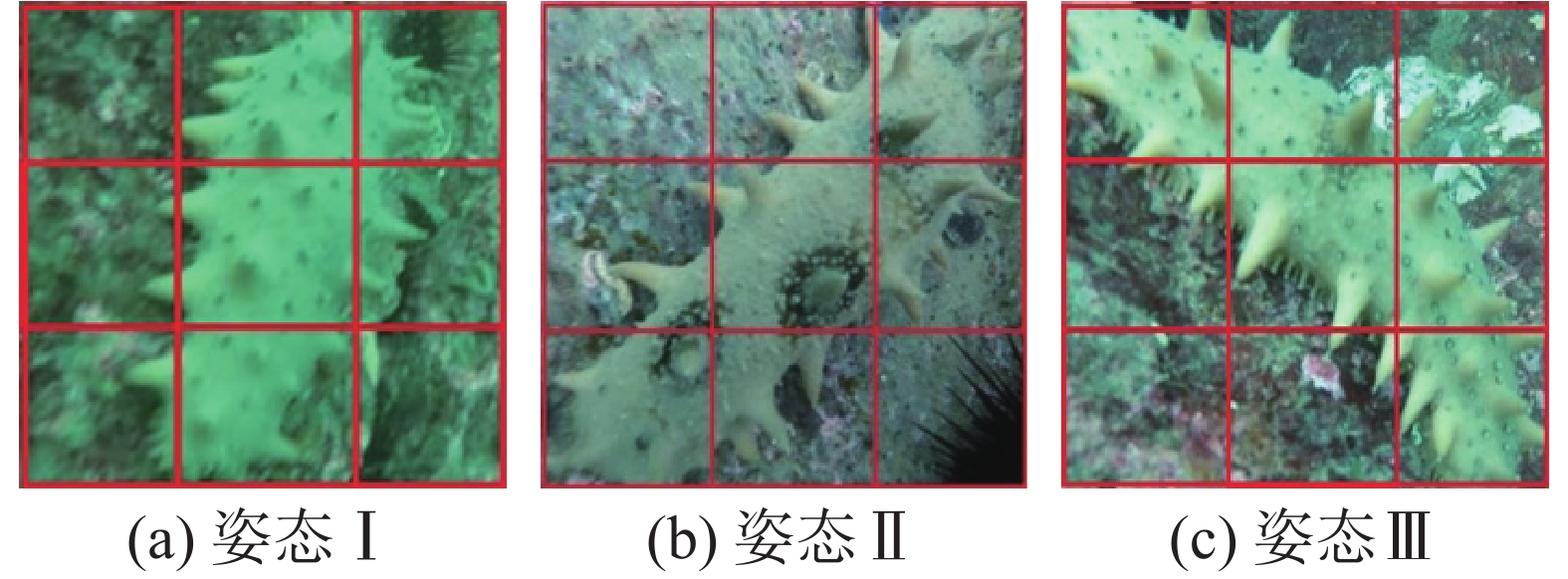Download: 图 5 海参目标选点原理图 Fig. 5 The target selection principle of a sea cucumber

 ${D_{ij}} = \left\| {x_{ij}^{} - x_{22}^{}} \right\| + \left\| {x_{4 - i,4 - j}^{} - x_{22}^{}} \right\|$ (15)

2.2 尺度和位置估计方法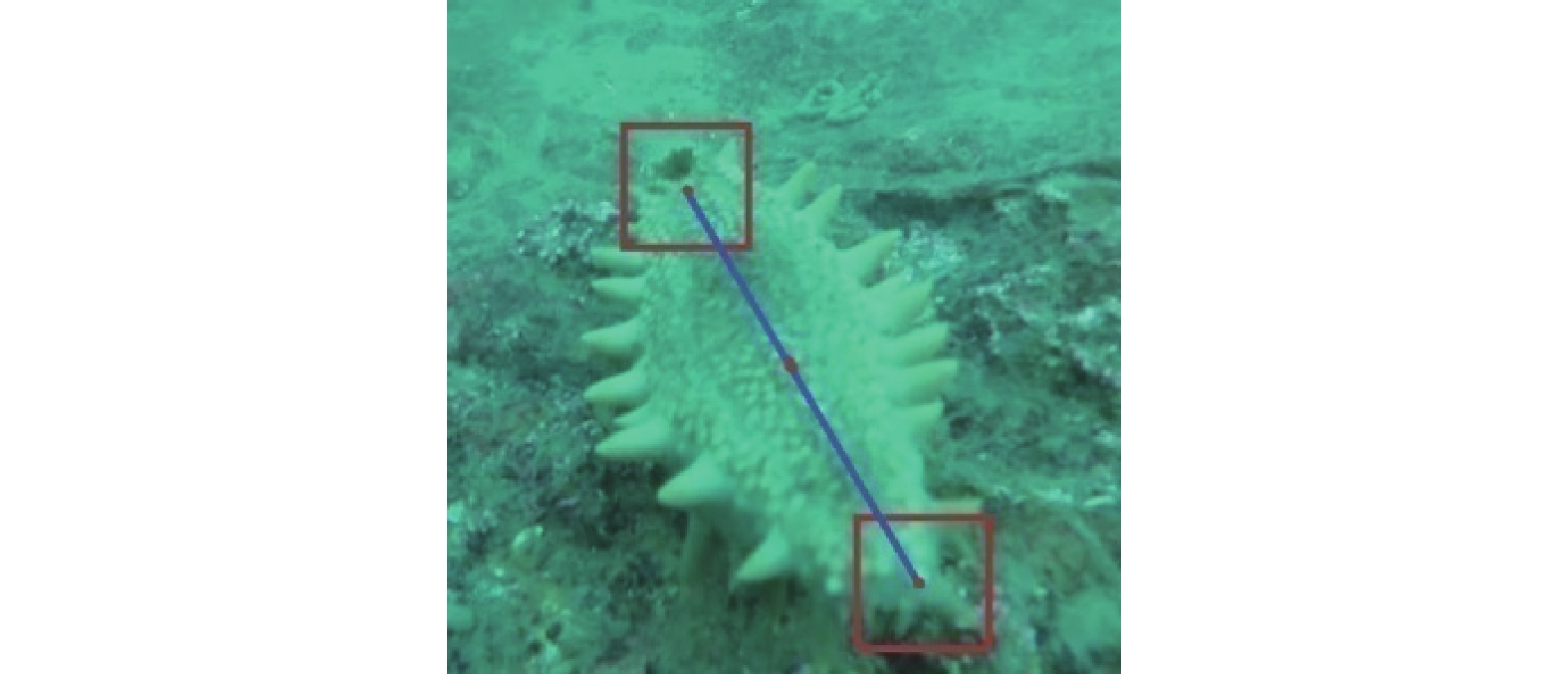Download: 图 6 海参尺度和位置估计示意 Fig. 6 Schematic representation of the scale and location estimation for a sea cucumber

 $p_{}^t = \frac{{p_A^t + p_B^t}}{2}$ (16)

 $S = \frac{{\left\| {p_A^t - p_B^t} \right\|}}{{\left\| {p_A^1 - p_B^1} \right\|}}$ (17)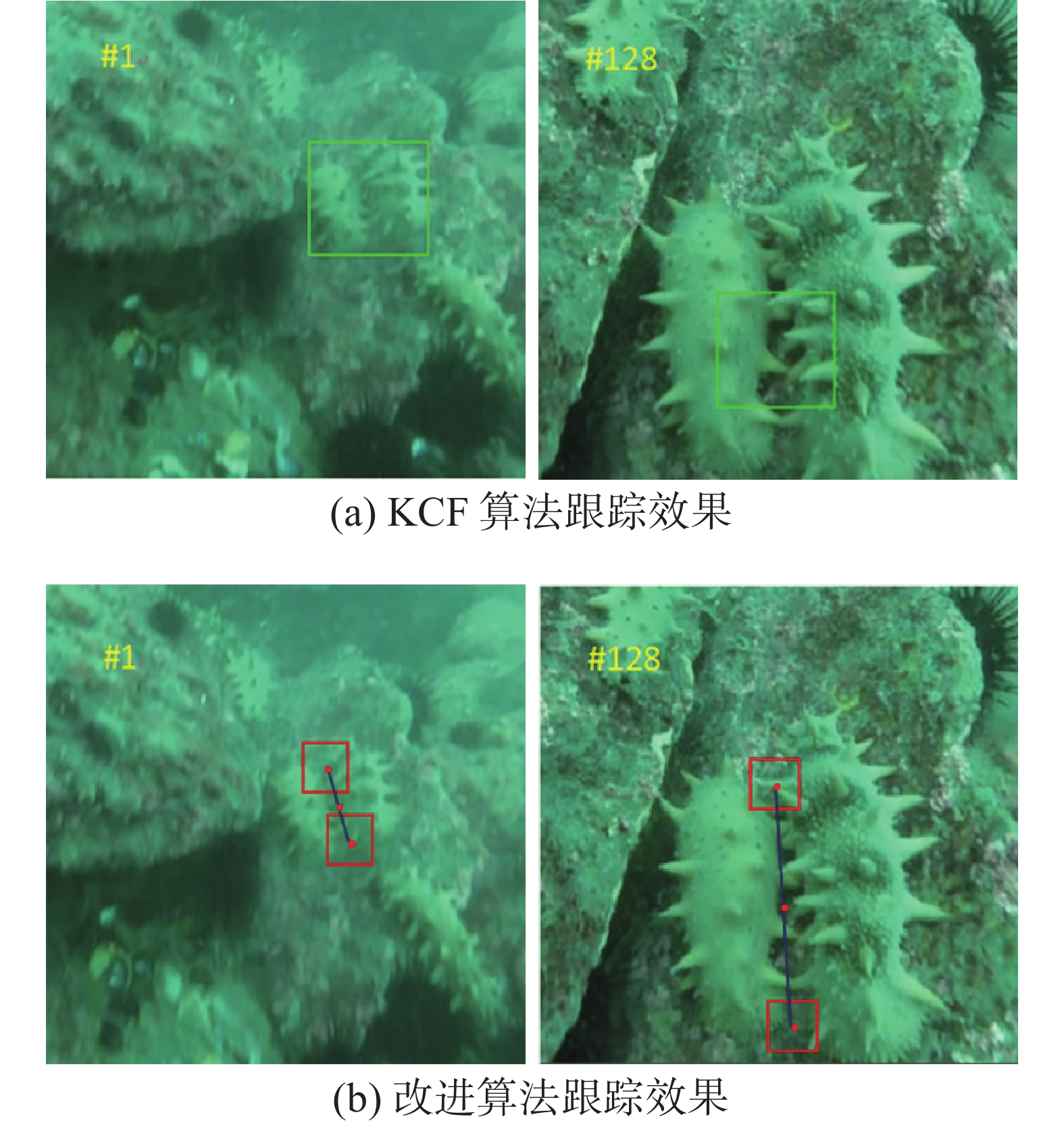Download: 图 7 追踪效果的比较 Fig. 7 Comparison of the tracking results
2.3 改进算法流程

1)当 $t = 1$ 时，根据初始条件计算目标位置 $p_A^1$ $p_B^1$ ，并截取图像块 ${x_1}$ ${x_2}$ ，通过式(3)计算得出基准输出样本 ${y_1}$ ${y_2}$ ；通过式(9)求核函数的傅氏变换 $\hat \kappa \left( {{x_1},{x_1}} \right)$ $\hat \kappa \left( {{x_2},{x_2}} \right)$ ，并求输出样本的傅氏变换 ${\hat y_1}$ ${\hat y_2}$ 。通过式(8)分别求解滤波器 ${\hat \alpha _1}$ ${\hat \alpha _2}$

2)当 $t > 1$ 时，在当前帧图像位置 $p_A^{t - 1}$ $p_B^{t - 2}$ 截取图像块 $\hat {\textit{z}}_1^t$ $\hat {\textit{z}}_2^t$ ，并通过式(9)求核函数的傅氏变换 $\hat \kappa \left( {x_1^t,{\textit{z}}_1^t} \right)$ $\hat \kappa \left( {x_2^t,{\textit{z}}_2^t} \right)$ ；通过式(11)计算响应面 $f_1^t$ $f_2^t$ ，并求响应面最大值得到两跟踪目标位置 $p_A^t$ $p_B^t$ ；通过式(14)求出目标的中心位置 $p_{}^t$ ，通过式(15)求出目标尺度因子 ${s^t}$ ；通过式(12)更新滤波器 $\hat \alpha _1^{t + 1}$ $\hat \alpha _2^{t + 1}$ ，并通过(13)更新目标外观模型 $\hat x_1^{t + 1}$ $\hat x_2^{t + 1}$

3 实试验结果分析表 1 视频参数 Tab.1 Video parameters
3.1 定性分析

5种对比算法中只有本文算法和DSST算法具有尺度估计环节，另外3种算法KCF、CSK和STC均采用固定尺度跟踪。图8中7组视频的共同特点是：目标尺度变化大，且由于水下特殊的成像环境导致图像质量较差。从跟踪结果可以看出：KCF、CSK和STC 3种算法追踪效果较差，而本文算法和DSST算法跟踪效果较好，可见对于尺度变化大的应用来说，尺度估计环节非常重要。图8中视频1~7代表7组视频系列实验比较结果。Download: 图 8 5种算法的定性比较 Fig. 8 Qualitative evaluation of the five algorithms
3.2 定量分析

1)跟踪速度表 2 平均每秒运行帧数 Tab.2 The average operation frames

2)距离精度

 $D = \frac{{n(d \leqslant {d_0})}}{n}$ (18)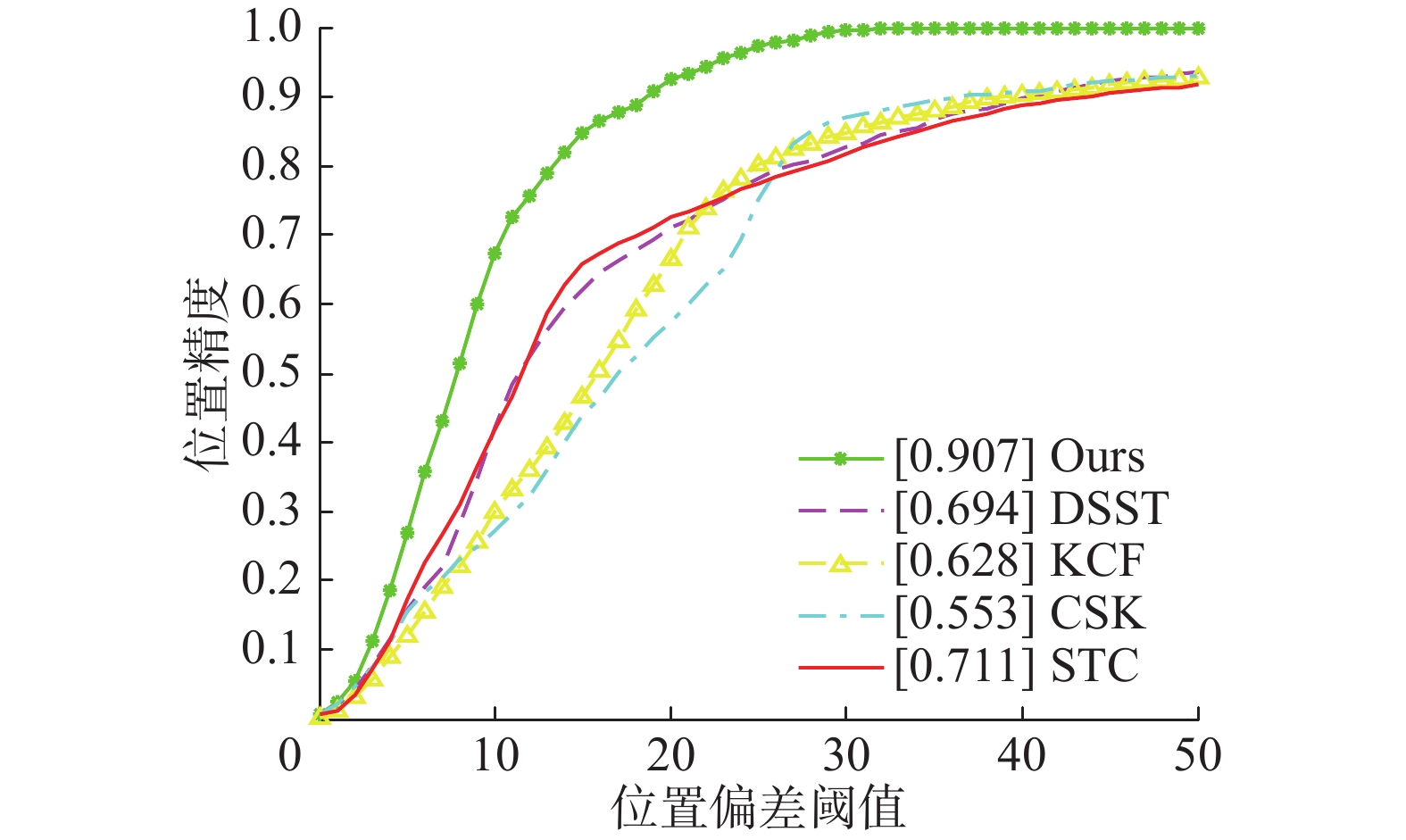Download: 图 9 在7个序列上的综合精确度曲线 Fig. 9 Precision plot over all the seven sequences表 3 平均精度 Tab.3 Average precision

3)跟踪成功率

 ${\rm{SR}} = \frac{{n(s \geqslant {s_0})}}{n}$ (19)

 $s = \frac{{{R_r} \cap {R_t}}}{{{R_r} \cup {R_t}}}$ (20)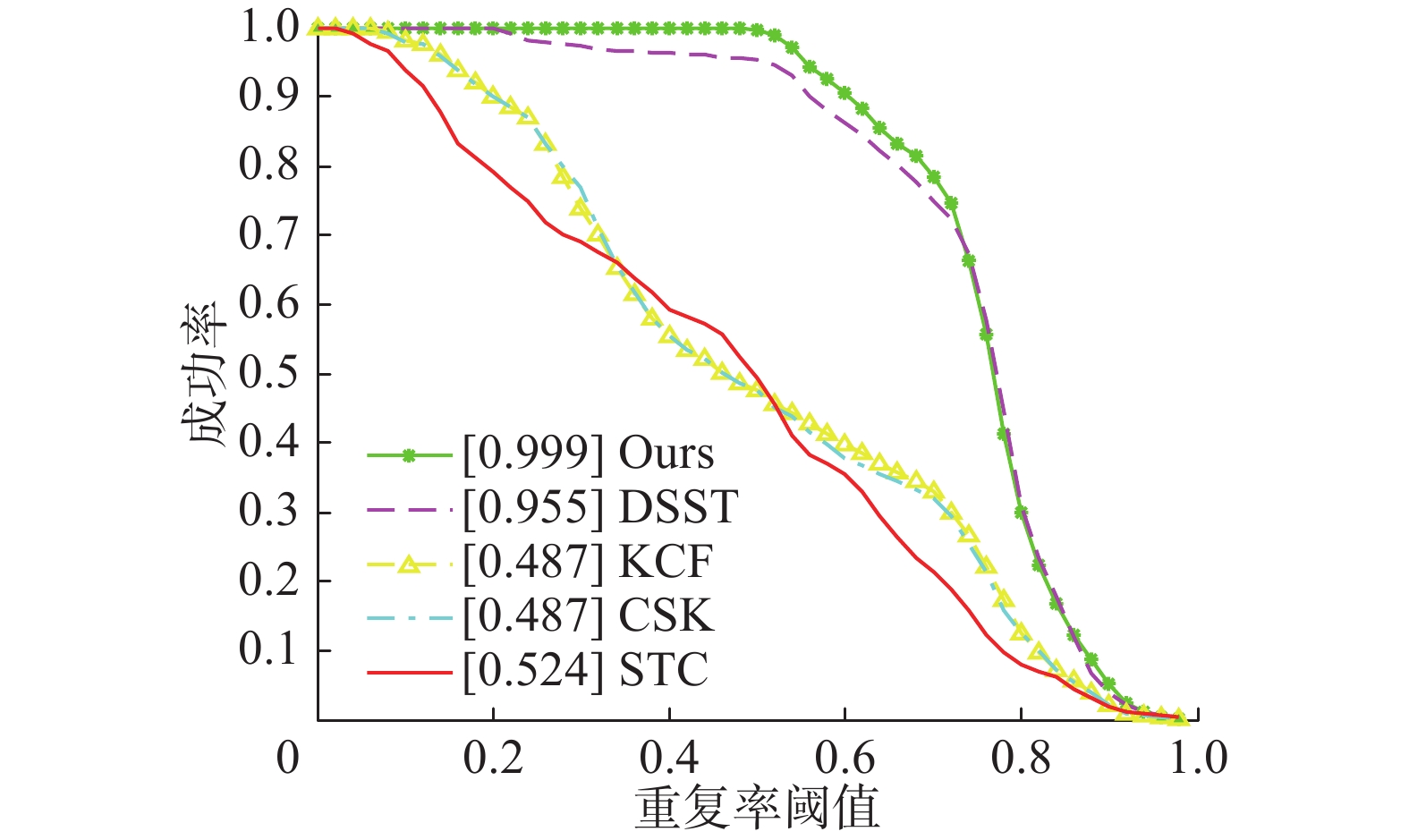Download: 图 10 在7个序列上的综合成功率曲线 Fig. 10 Success plots over all the seven sequences表 4 平均成功率 Tab.4 Average success rate
4 结束语

  郭传鑫, 李振波, 乔曦, 等. 基于融合显著图与GrabCut算法的水下海参图像分割[J]. 农业机械学报, 2015(S1): 147-152. GUO Chuanxin, LI Zhenbo, QIAO Xi, et al. Image segmentation of underwater sea cucumber using GrabCut with saliency map[J]. Transactions of the Chinese society for agricultural machinery, 2015(S1): 147-152. DOI:10.6041/j.issn.1000-1298.2015.S0.025 (0)  唐旭东, 庞永杰, 张赫, 等. 基于单目视觉的水下机器人管道检测[J]. 机器人, 2010, 32(5): 592-600. TANG Xudong, PANG Yongjie, ZHANG He, et al. Underwater pipeline detection by AUV based on monocular vision[J]. Robot, 2010, 32(5): 592-600. (0)  曾文静, 徐玉如, 万磊, 等. 自主式水下机器人的光视觉管道探测跟踪系统[J]. 上海交通大学学报, 2012, 46(2): 178-183, 189. ZENG Wenjing, XU Yuru, WAN Lei, et al. Robotics vision-based system of autonomous underwater vehicle for an underwater pipeline tracker[J]. Journal of Shanghai Jiaotong University, 2012, 46(2): 178-183, 189. (0)  吴利红, 许文海, 王利鹏. AUV水下终端对接目标识别与定位技术[J]. 大连海事大学学报, 2014, 40(2): 81-85. WU Lihong, XU Wenhai, WANG Lipeng. Dock position and pose estimation algorithm for AUV underwater terminal docking[J]. Journal of Dalian Maritime University, 2014, 40(2): 81-85. DOI:10.3969/j.issn.1006-7736.2014.02.020 (0)  WU Yi, LIM J, YANG M H. Online object tracking: a benchmark[C]//Proceedings of 2013 IEEE Conference on Computer Vision and Pattern Recognition. Portland, OR, USA, 2013: 2411–2418. (0)  BOLME D S , BEVERIDGE J R , DRAPER B A , et al. Visual object tracking using adaptive correlation filters[C]//The Twenty-Third IEEE Conference on Computer Vision and Pattern Recognition, CVPR 2010.San Francisco, CA, USA, 2010:13-18. (0)  HENRIQUES J F, CASEIRO R, MARTINS P, et al. Exploiting the circulant structure of tracking-by-detection with kernels[C]//Proceedings of the 12th European Conference on Computer Vision Computer Vision-ECCV 2012. Florence, Italy, 2012: 702–715. (0)  HENRIQUES J F, CASEIRO R, MARTINS P, et al. High-speed tracking with kernelized correlation filters[J]. IEEE transactions on pattern analysis and machine intelligence, 2015, 37(3): 583-596. DOI:10.1109/TPAMI.2014.2345390 (0)  杨德东, 蔡玉柱, 毛宁, 等. 采用核相关滤波器的长期目标跟踪[J]. 光学精密工程, 2016, 24(8): 2037-2049. YANG Dedong, CAI Yuzhu, MAO Ning, et al. Long-term object tracking based on kernelized correlation Filters[J]. Optics and precision engineering, 2016, 24(8): 2037-2049. (0)  ZHANG Kaihua, ZHANG Lei, LIU Qingshan, et al. Fast visual tracking via dense spatio-temporal context learning[C]//Proceedings of the 13th European Conference on Computer Vision. Zurich, Switzerland, 2014: 127–141. (0)  张雷, 王延杰, 刘艳滢, 等. 基于相关滤波器的视觉目标跟踪方法[J]. 光电子·激光, 2015, 26(7): 1349-1357. ZHANG Lei, WANG Yanjie, LIU Yanying, et al. Visual object tracking algorithm based on correlation filters[J]. Journal of optoelectronics laser, 2015, 26(7): 1349-1357. (0)  段伟伟, 杨学志, 方帅, 等. 分块核化相关滤波目标跟踪[J]. 计算机辅助设计与图形学学报, 2016, 28(7): 1160-1168. DUAN Weiwei, YANG Xuezhi, FANG Shuai, et al. Block-based kernelized correlation filters object tracking[J]. Journal of computer-aided design and computer graphics, 2016, 28(7): 1160-1168. DOI:10.3969/j.issn.1003-9775.2016.07.016 (0)  邢运龙, 李艾华, 崔智高, 等. 改进核相关滤波的运动目标跟踪算法[J]. 红外与激光工程, 2016, 45(S1): 214-221. XING Yunlong, LI Aihua, CUI Zhigao, et al. Moving target tracking algorithm based on improved Kernelized correlation filter[J]. Infrared and laser engineering, 2016, 45(S1): 214-221. (0)  DANELLJAN M, HÄGER G, KHAN F S, et al. Accurate scale estimation for robust visual tracking[C]//Proceedings of the British Machine Vision Conference. Sweden, 2014: 65.1–65.11. (0)  JIA Xu, LU Huchuan, YANG M H. Visual tracking via adaptive structural local sparse appearance model[C]//Proceedings of 2012 IEEE Conference on Computer Vision and Pattern Recognition. Providence, RI, USA, 2012: 1822–1829. (0)  ZHONG Wei, LU Huchuan, YANG M H. Robust object tracking via sparsity-based collaborative model[C]//Proceedings of 2012 IEEE Conference on Computer Vision and Pattern Recognition. Providence, RI, USA, 2012: 1838–1845. (0)  程子一, 刘志林. 改进的核相关滤波算法在自航模动态目标跟踪应用[J]. 应用科技, 2019, 46(1): 36-42. CHENG Ziyi, LIU Zhilin． Application of improved kernel correlation filtering algorithm in small ship dynamic target tracking[J]. Applied science and technology, 2019,46(1): 36-42． (0)  朱齐丹, 韩瑜, 蔡成涛. 全景视觉非线性核相关滤波目标跟踪技术[J]. 哈尔滨工程大学学报, 2018, 39(7): 1220-1226. ZHU Qidan, HAN Yu, CAI Chengtao. Omni-directional visual object tracking using nonlinear kernelized correlation filters[J]. Journal of Harbin Engineering University, 2018, 39(7): 1220-1226. (0)  诸小熊, 江加和. 基于核相关滤波器的目标跟踪算法[J]. 应用科技, 2017, 44(3): 48-53. ZHU Xiaoxiong, JIANG Jiahe. Visual tracking algorithm based on kernelized correlation filters[J]. Applied science and technology, 2017, 44(3): 48-53. (0)